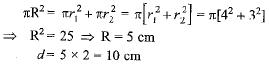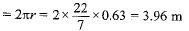Courses

# MCQ : Areas Related To Circles - 1

## 10 Questions MCQ Test Mathematics (Maths) Class 10 | MCQ : Areas Related To Circles - 1

Description
This mock test of MCQ : Areas Related To Circles - 1 for Class 10 helps you for every Class 10 entrance exam. This contains 10 Multiple Choice Questions for Class 10 MCQ : Areas Related To Circles - 1 (mcq) to study with solutions a complete question bank. The solved questions answers in this MCQ : Areas Related To Circles - 1 quiz give you a good mix of easy questions and tough questions. Class 10 students definitely take this MCQ : Areas Related To Circles - 1 exercise for a better result in the exam. You can find other MCQ : Areas Related To Circles - 1 extra questions, long questions & short questions for Class 10 on EduRev as well by searching above.
QUESTION: 1

### If the area of a circle is 154 cm2, then its perimeter is

Solution:

Given area of circle=154
⇒area of circle=πr²
=22/7 ×7×7.
=154
so radius of the circle=7cm
perimeter of circle=2πr
=2 ×22/7×7
=44cm
⇒perimeter of the circle=44 cm.

QUESTION: 2

### The radii of two circles are 4 cm and 3 cm respectively. The diameter of the circle having area equal to the sum of the areas of the two circles (in cm)

Solution:QUESTION: 3

### If the circumference of a circle and the perimeter of a square are equal, the

Solution:
QUESTION: 4

The perimeter (in cm) of a square circumscribing a circle of radius a cm, is

Solution:

Side of a square circumscribing a circle of radius a cm = diameter of circle = 2a cm
∴ Perimeter of the square = 4 x 2a = 8a cm

QUESTION: 5

The perimeter of a circle is equal to that of a square, then the ratio of their areas is​

Solution:
QUESTION: 6

If the area of a circle is numerically equal to twice its circumference, then the diameter of the circle is

Solution:

πr2 - 2πr x 2 ⇒ r = 4 ⇒ 2r = 8 units

QUESTION: 7

The area of the circle that can be inscribed in a square of side 6 cm is

Solution:
QUESTION: 8

The diameter of a wheel is 1.26 m. The distance travelled in 500 revolutions is

Solution:

Radius of the wheel = 1.26/2 = 0.63 m
Distance travelled in one revolution∴ Distance travelled in 500 revolutions
= 500 x 3.96 = 1980 m

QUESTION: 9

The radius of a circle whose circumference is equal to the sum of the circumferences of the two circles of diameters 36 cm and 20 cm is​

Solution:

Diameter of first circle = d1 = 36 cm
Diameter of second circle = d2 = 20 cm
∴ Circumference of first circle = πd1 = 36π cm
Circumference of second circle = πd2 = 20π cm
Now, we are given that,
Circumference of circle = Circumference of first circle + Circumference of second circle
πD = πd1 + πd2
⇒ πD = 36π + 20π
⇒ πD = 56π ⇒ D = 56
⇒ Radius = 56/2 = 28 cm

QUESTION: 10

If the sum of the circumferences of two circles with radii R1 and R2 is equal to the circumference of a circle of radius R, then

Solution:

2π R1 + 2πR2 = 2πR
⇒ R1 + R2 = R.